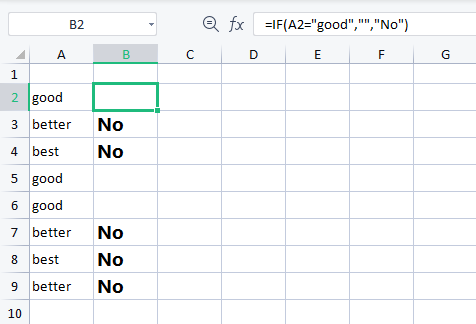# How to use if cell conatins formula in excel

August 1, 2022
1.3K Views
0

A free Office suite fully compatible with Microsoft OfficeYou may utilise your data in helpful ways with the use of a variety of Excel formulae. You may, for instance, receive a result depending on whether or not a cell satisfies a set of requirements. We'll concentrate on the if cell contains function right now.

Return a value if the cell has any values:

In this case, you have the option to return values based on whether a cell has any values at all. As an illustration, we will determine whether or not the A1 cell is empty and then return a value in accordance with the outcome.

Use the following formula to choose the output cell: =IF(cell>, value to return, ).

In our case, cell A2 will be the one we wish to check, and the result will be No. You would modify the expression to =IF(A2>, No,  in this case).

The formula will return No in the output cell since the A2 cell isn't empty. The output cell will stay blank if the cell you're examining is empty.

Return a value if a cell contains either text or a number:

Look for a text:

If the target cell contains any text or numbers, you may use the formula below to return a certain value. The opposing data types will be ignored by the calculation.

Select the output cell and enter the following formula to determine if a cell includes text:=IF(ISTEXT(cell), value_to_return, ).

In our example, cell A2 will be the one we wish to check, and yes will be the result. In this case, the expression would be changed to=IF(ISTEXT(A2), Yes, ).

The formula will output Yes since the A2 cell contains text and not a number or a date or time.

Look for a date or a number:

Use the following formula to determine if a cell contains a number or date:

=IF(ISNUMBER(cell), value_to_return, ).

In our case, cell D2 will be the one we wish to check, and the result will be Yes. In this case, the formula would be changed to =IF(ISNUMBER(D2), Yes, ).

The formula will return Yes into the output cell since the D2 cell does have a numeric value in it rather than text.

Return a value if  cell contains a certain piece of text.

Use the formula below to locate a cell that has a particular string of text in it.

Use the formula =IF(cell=text, value_to_return, ) to choose the output cell.

The text we're looking for in our example is example, the cell we want to check is A2, and the return value is Yes. In this case, the expression would be changed to

=IF(A2=example, Yes, ).

The formula will return Yes into the output cell since the A2 field does include the text example.

Return a value if  cell contains a certain piece of text (case-sensitive):

Use the formula below to locate a cell that has a particular string of text in it. Since this version is case-sensitive, only cells that exactly match the value will be returned.

Use the following formula to choose the output cell:

=IF(EXACT(cell,case sensitive text), value_to_return, ).

The text we're looking for in our example is EXAMPLE, the cell we want to check is A2, and the return value is Yes. In this case, the expression would be changed to

=IF(EXACT(A2,EXAMPLE), Yes, ).

The formula will return Yes into the output cell since the A2 field does include the phrase EXAMPLE in the correct situation.

Return a value if the cell does not contain any specific content:

Use this formula to locate cells that don't contain a certain text.

Use the following formula to choose the output cell:=IF(cell=text, , value_to_return).

The text we're looking for in our example is example, the cell we want to check is A2, and the return value is No. In this case, the formula would be changed to =IF(A2=example, , No).

The formula will return a blank cell since the A2 cell does include the word example in its entirety. However, other cells give the output cell a No response.